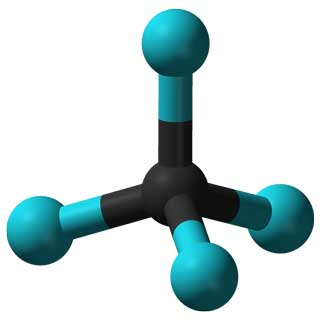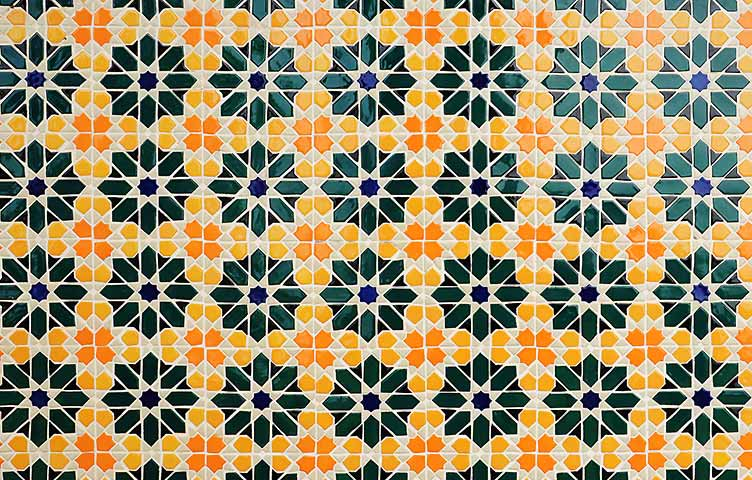## Глосарій

Виберіть одне з ключових слів ліворуч ...

# Transformations and SymmetrySymmetry Groups and Wallpapers

Час читання: ~25 min

Some shapes have more than one symmetry – let’s have a look at the square as a simple example.You have already shown above that a square has axes of reflection.

It also has rotational symmetry by °, ° and °.

And finally, we can think about “doing nothing” as another special kind of symmetry – because the result is (obviously) the same as before. This is sometimes called the identity.

In total, we have found different “symmetries of the square”.

Now we can actually start doing some arithmetic with these symmetries. For example, we can add two symmetries to get new ones:+=+=Whenever you add two symmetries of a square, you get a new one. Here is a “symmetry calculator” where you can try it yourself:

+
=
×

Spend some time playing around with the symmetry calculator, and try to find any patterns. Can you complete these observations?

• Adding two rotations will always give (or the identity).
• Adding two reflections will always give (or the identity).
• Adding the same two symmetries in the opposite order result.

1. Adding two symmetries/integers always gives another symmetry/integer:+=12+7=19
Continue++=++4+2+5=4+2+5
Continue
3. Every symmetry/integer has an inverse, another symmetry/integer which, when added, gives the identity:+=4+–4=0
Continue

In mathematics, any collection that has these properties is called a group. Some groups (like the symmetries of a square) only have a finite number of elements. Others (like the integers) are infinite.

In this example, we started with the eight symmetries of the square. In fact, every geometric shape has its own symmetry group. They all have different elements, but they always satisfy the three rules above.

Groups appear everywhere in mathematics. The elements can be numbers or symmetries, but also polynomials, permutations, matrices, functions … anything that obeys the three rules. The key idea of group theory is that we are not interested in the individual elements, just in how they interact with each other.

For example, the symmetry groups of different molecules can help scientists predict and explain the properties of the corresponding materials.

Groups can also be used to analyse the winning strategy in board games, the behaviour of viruses in medicine, different harmonies in music, and many other concepts…The properties of the CCl4 molecule (left) and the Adenovirus (right) are determined by their symmetries.

## Wallpaper Groups

In the previous sections we saw two different kinds of symmetry corresponding to two different transformations: rotations and reflections. But there is also a symmetry for the third kind of rigid transformation: .

Translational symmetry does not work for isolated objects like flowers or butterflies, but it does for regular patterns that extend into every direction:Hexagonal honyecombCeramic wall tiling

In addition to reflectional, rotational and translational symmetry, there even is a fourth kind: glide reflections. This is a combination of a reflection and a translation in the same direction as the axis of reflection.

A pattern can have more than one type of symmetry. And just like for squares, we can find the symmetry group of a pattern, which contains all its different symmetries.

These groups don’t tell you much about how the pattern looks like (e.g. its colours and shapes), just how it is repeated. Multiple different patterns can have the same symmetry group – as long are arranged and repeated in the same way.These two patterns have the same symmetries, even though they look very different. But symmetries are not about colours, or superficial shapes.These two patterns also have the same symmetries – even though they look more similar to the corresponding patterns on the left, than to each other.

It turns out that, while there are infinitely many possible patterns, they all have one of just 17 different symmetry groups. These are called the Wallpaper Groups. Every wallpaper group is defined by a combination of translations, rotations, reflections and glide reflections. Can you see the centers of rotation and the axes of reflection in these examples?Group 1 – P1
Only translationsGroup 2 – P2
Rotations of order 2, translationsGroup 3 – P3
Rotations of order 3 (120°), translationsGroup 4 – P4
Four rotations of order 2 (180°), translationsGroup 5 – P6
Rotations of order 2, 3 and 6 (60°), translationsGroup 6 – PM
Parallel axes of reflection, translationsGroup 7 – PMM
Perpendicular reflections, rotations of order 2, translationsGroup 8 – P4M
Rotations (ord 2 + 4), reflections, glide reflections, translationsGroup 9 – P6M
Rotations (ord 2 + 6), reflections, glide reflections, translationsGroup 10 – P3M1
Rotations of order 3, reflections, glide reflections, translationsGroup 11 – P31M
Rotations of order 3, reflections, glide reflections, translationsGroup 12 – P4G
Rotations (ord 2 + 4), reflections, glide reflections, translationsGroup 13 – CMM
Perpendicular reflections, rotations of order 2, translationsGroup 14 – PMG
Reflections, glide reflections, rotations of order 2, translationsGroup 15 – PG
Parallel glide reflections, translationsGroup 16 – CM
Reflections, glide reflections, translationsGroup 17 – PGG
Perpendicular glide reflections, rotations of order 2, translations

Unfortunately there is no simple reason why there are 17 of these groups, and proving it requires more advanced mathematics. Instead, you can try drawing your own repeated patterns for each of the 17 wallpaper groups:

#### Examples of other students’ drawingsThe wallpaper groups were all about flat, two-dimensional patterns. We can do something similar for three-dimensional patterns: these are called crystallographic groups, and there are 219 of them!

In addition to translations, reflections, rotations, and glide reflections, these groups include symmetries like glide planes and screw axes (think about the motion when unscrewing a bottle).Boron-Nitride has its molecules arranged in this crystal lattice, which has a three-dimensional symmetry group.

Archie